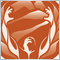• Übersicht
• Bewertungen (1)
• Diskussion (12)

# WD Gann Analysis

How you benefit form WD Gann Analysis Indicator?

• No chart scaling required, gann indicator use time and price movement to draw gann angles, so angles will not change the location when zooming the chart or switching between different time frames.

Watch this Video.

• Draw Correct Gann Angles for Forex, Gann Angles points and time input is not fixed so you can change the point and time value to draw the correct gann angles without changing the price quotes.

Watch this Video.

• Four types of gann angles will help you to draw any gann angles type over any time frame, you can draw weekly, monthly or calendar angles over the daily chart.

Also, it will help you to find the correct time factor for each instrument you trade and forecast future gann angles to trade.

Watch this Video.

• Custom gann angles will help you to draw additional gann angles from any swing high, low, or zero price, so you can find additional support and resistance angles.

Watch this Video.

• Multi Angles will save your time and draw 10 gann angles based on the time and price inputs, so you can easily identify the gann angles type when analysing the chart.
• Gann percentages will help you to find the relation between the high and low price and forecast the future levels to trade.

Watch video for Gann Percentage Training lessons

Watch video for Gann Percentages Rules

• Cutting gann angles and percentages using the cuter icon will protect you from misjudging about the levels you are watching.
• WD Gann Analysis VS MT4 Gann Fan

Watch this Video

Indicator Settings

The main indicator setting is Gann Analysis Mode setting where you will see two options to choose:

• Angle – choose this option to draw Gann Angles on the chart.
• Percentage – choose this option to draw Gann Percentage Level on the chart.

Angles Settings

• Angle Points: the value of angle point movement.
• Angle Time: the value of angles time movement.
• Angle Type: there are four types of angles we can draw: Daily, Calendar, Weekly and Monthly Angles.
• Angle Mode: there are Three modes for Angles:
• SingleAngle: to draw one WD GANN Angle line.
• MultiAngle: to draw 10 WD GANN Angles line starting from Angle point and time value.
• Custom Angle: to draw One WD GANN Angle from a specific Price.

Percentage Settings

• Percentage Level: the value of the Percentage Level we need to draw on the chart.
• Percentage Mode: there are four options to choose:
• SinglePercent to draw one Percentage Level.
• MultiPercent to draw 4 Percentage Levels on the chart (this will multiply the Percentage Level to 4 levels).
• FullPercent to draw full WD GANN Percentage Levels.

Please watch Video explaining all above setting and how to use32
2017.01.24 12:31

I read about this indicator in the fall of 2016 and because I had read a lot about WD Gann I bought it for a whole year.

What AMD has done here is magnificent, it is simple to use, effective and enjoyable.

I recommend using the "Gann Analysis" indicator, if you have patience for life.

Sincer - HlyGson